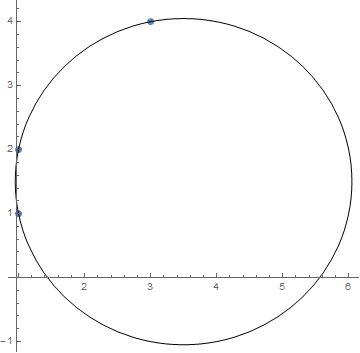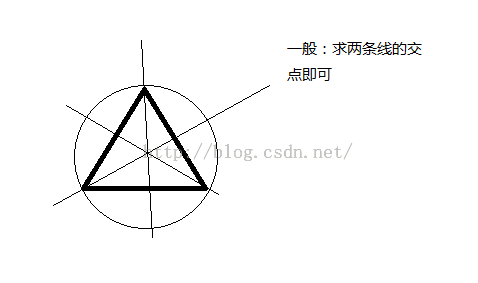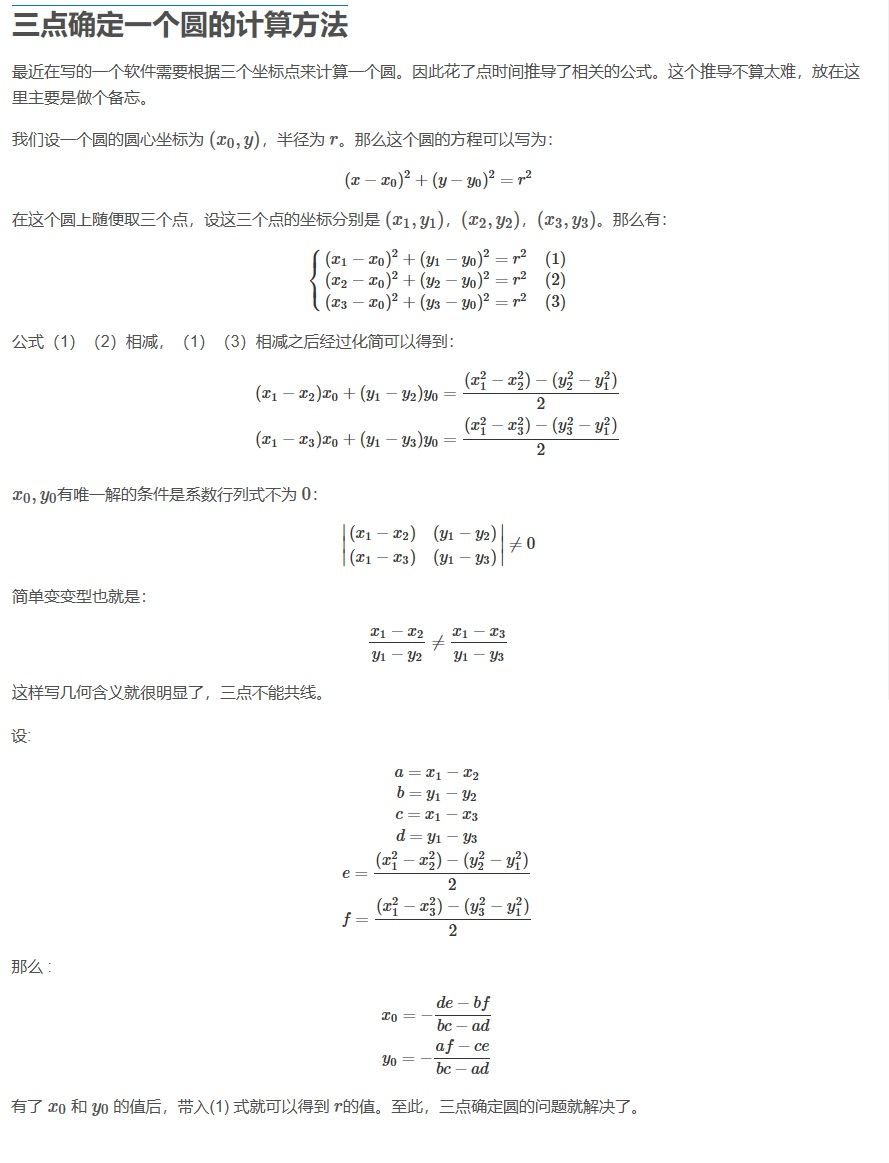• ## 三个点确定一个圆

千次阅读 2017-10-01 08:17:06
首先假设圆心为(x0, y0)，半径为r。则这个可以表示为： ...假设知道的三个点为(x1, y1)，(x2,y2)，(x3,y3)。那么： (x1−x0)2+(y1−y0)2=r2(x1 - x0)^2+(y1 - y0)^2 = r^2 (x2−x0)2+(y2−y0)2=r2(x2 - x0)^2+
首先假设圆心为(x0, y0)，半径为r。则这个圆可以表示为：

(x−x0)2+(y−y0)2=r2​
$(x - x0)^2+(y - y0)^2 = r^2​$
假设知道的三个点为(x1, y1)，(x2,y2)，(x3,y3)。那么：

(x1−x0)2+(y1−y0)2=r2
$(x1 - x0)^2+(y1 - y0)^2 = r^2$

(x2−x0)2+(y2−y0)2=r2
$(x2 - x0)^2+(y2 - y0)^2 = r^2$

(x3−x0)2+(y3−y0)2=r2
$(x3 - x0)^2+(y3 - y0)^2 = r^2$
消去

r2
$r^2$后变成

(x1−x0)2+(y1−y0)2=(x2−x0)2+(y2−y0)2
$(x1 - x0)^2+(y1 - y0)^2 = (x2 - x0)^2+(y2 - y0)^2$

(x1−x0)2+(y1−y0)2=(x3−x0)2+(y3−y0)2
$(x1 - x0)^2+(y1 - y0)^2 = (x3 - x0)^2+(y3 - y0)^2$
将未知量x0,y0移到左边就变为

(x1−x2)x0+(y1−y2)y0=(x12−x22)+(y12−y22)2
$(x1 - x2)x0 + (y1 - y2)y0 = \frac{(x1^2 - x2^2) + (y1^2 - y2^2)}{2}$

(x1−x3)x0+(y1−y3)y0=(x12−x32)+(y12−y32)2
$(x1 - x3)x0 + (y1 - y3)y0 = \frac{(x1^2 - x3^2) + (y1^2 - y3^2)}{2}$
首先我们要确定这个方程是不是有解，很简单，即判断行列式

∣∣∣(x1−x2)(x1−x3)(y1−y2)(y1−y3)∣∣∣≠0
$\left| \begin{array} ((x1 - x2) & (y1 - y2) \\(x1 - x3) & (y1 - y3) \end{array} \right | \neq 0$
设两个常量a1,a2

a1=(x12−x22)+(y12−y22)2
$a1 = \frac{(x1^2 - x2^2) + (y1^2 - y2^2)}{2}$

a2=(x12−x32)+(y12−y32)2
$a2 = \frac{(x1^2 - x3^2) + (y1^2 - y3^2)}{2}$
那么

x0=(y1−y2)a2−(y1−y3)a1(y1−y2)(x1−x3)−(x1−x2)(y1−y3)
$x0 = \frac{(y1 - y2)a2 - (y1 - y3)a1}{(y1 - y2)(x1 - x3)-(x1 - x2)(y1-y3)}$

y0=(x1−x3)a1−(x1−x2)a2(y1−y2)(x1−x3)−(x1−x2)(y1−y3)
$y0 = \frac{(x1 - x3)a1 - (x1 - x2)a2}{(y1 - y2)(x1 - x3)-(x1 - x2)(y1-y3)}$
最后代码如下
import numpy as np
import matplotlib.pyplot as plt
from matplotlib.patches import Circle
#www.coordinate.wang
def circle(x1, y1, x2, y2, x3, y3):
a = x1 - x2
b = y1 - y2
c = x1 - x3
d = y1 - y3
a1 = ((x1 * x1 - x2 * x2) + (y1 * y1 - y2 * y2)) / 2.0
a2 = ((x1 * x1 - x3 * x3) + (y1 * y1 - y3 * y3)) / 2.0
theta = b * c - a * d;
if abs(theta) < 1e-7:
return -1
x0 = (b * a2 - d * a1) / theta;
y0 = (c * a1 - a * a2) / theta;
r = np.sqrt(pow((x1 - x0), 2)+pow((y1 - y0), 2))
fig = plt.figure()
cir = Circle(xy = (x0, y0), radius = r, alpha=0.4)
plt.axis('scaled')
plt.axis('equal')
plt.plot(x1,y1,'r*',x2,y2,'r*',x3,y3,'r*')
plt.show()

circle(0, 0, 0, 1, 1, 0)
结果为展开全文python 算法
• ## 三点确定一个圆的计算方法

万次阅读 多人点赞 2016-10-22 13:40:51
三点确定一个圆的计算方法最近在写的一个软件需要根据三个坐标来计算一个圆。因此花了时间推导了相关的公式。这个推导不算太难，放在这里主要是做个备忘。我们设一个圆的圆心坐标为 (x0,y)(x_0, y)，半径为 rr。...
三点确定一个圆的计算方法
最近在写的一个软件需要根据三个坐标点来计算一个圆。因此花了点时间推导了相关的公式。这个推导不算太难，放在这里主要是做个备忘。
我们设一个圆的圆心坐标为

(x0,y)
$(x_0, y)$，半径为

r
$r$。那么这个圆的方程可以写为：
(x−x0)2+(y−y0)2=r2
(x - x_0)^2 + (y - y_0)^2 = r^2

在这个圆上随便取三个点，设这三个点的坐标分别是

(x1,y1)
$(x_1, y_1)$，

(x2,y2)
$(x_2, y_2)$，

(x3,y3)
$(x_3, y_3)$。那么有：

⎧⎩⎨⎪⎪(x1−x0)2+(y1−y0)2=r2(x2−x0)2+(y2−y0)2=r2(x3−x0)2+(y3−y0)2=r2(1)(2)(3)

\begin{cases}
(x_1 - x_0)^2 + (y_1-y_0)^2 = r^2 & (1)\\
(x_2 - x_0)^2 + (y_2-y_0)^2 = r^2 & (2)\\
(x_3 - x_0)^2 + (y_3-y_0)^2 = r^2 & (3)
\end{cases}

公式（1）（2）相减，（1）（3）相减之后经过化简可以得到：

(x1−x2)x0+(y1−y2)y0=(x21−x22)−(y22−y21)2(x1−x3)x0+(y1−y3)y0=(x21−x23)−(y23−y21)2

(x_1 - x_2) x_0 + (y_1- y_2) y_0 = \frac{(x_1^2-x_2^2)-(y_2^2-y_1^2)}{2}\\
(x_1 - x_3) x_0 + (y_1- y_3) y_0 = \frac{(x_1^2-x_3^2)-(y_3^2-y_1^2)}{2}\\

x0,y0
$x_0,y_0$有唯一解的条件是系数行列式不为

0
$0$：
∣∣∣(x1−x2)(x1−x3)(y1−y2)(y1−y3)∣∣∣≠0
\begin{vmatrix}
(x_1 - x_2) & (y_1- y_2) \\
(x_1 - x_3) & (y_1- y_3)
\end{vmatrix} \neq 0

简单变变型也就是：

x1−x2y1−y2≠x1−x3y1−y3

\frac{x_1 - x_2}{y_1-y_2} \neq \frac{x_1 - x_3}{y_1-y_3}

这样写几何含义就很明显了，三点不能共线。
设:

a=x1−x2b=y1−y2c=x1−x3d=y1−y3e=(x21−x22)−(y22−y21)2f=(x21−x23)−(y23−y21)2

a = x_1-x_2\\
b = y_1-y_2\\
c = x_1-x_3\\
d = y_1-y_3\\
e = \frac{(x_1^2-x_2^2)-(y_2^2-y_1^2)}{2}\\
f = \frac{(x_1^2-x_3^2)-(y_3^2-y_1^2)}{2}

那么 :

x_0 = -\frac{d e-b f}{b c-a d}\\
y_0 = -\frac{a f-c e}{b c-a d}

有了

x0
$x_0$ 和

y0
$y_0$ 的值后，带入(1) 式就可以得到

r
<script type="math/tex" id="MathJax-Element-17">r</script>的值。至此，三点确定圆的问题就解决了。
下面是个 C++ 代码（用到了Qt 的 QPointF 类型）：
    #include <math.h>
#include <limits>
#include <QPoint>
#include <QDebug>
QPointF tcircle(QPointF pt1, QPointF pt2, QPointF pt3, double &radius)
{
double x1 = pt1.x(), x2 = pt2.x(), x3 = pt3.x();
double y1 = pt1.y(), y2 = pt2.y(), y3 = pt3.y();
double a = x1 - x2;
double b = y1 - y2;
double c = x1 - x3;
double d = y1 - y3;
double e = ((x1 * x1 - x2 * x2) + (y1 * y1 - y2 * y2)) / 2.0;
double f = ((x1 * x1 - x3 * x3) + (y1 * y1 - y3 * y3)) / 2.0;
double det = b * c - a * d;
if( fabs(det) < 1e-5)
{
return QPointF(0,0);
}

double x0 = -(d * e - b * f) / det;
double y0 = -(a * f - c * e) / det;
radius = hypot(x1 - x0, y1 - y0);
return QPointF(x0, y0);
}
用 {1, 1}, {1, 2}, {3, 4} 这三个点测试了一下。结果没有问题。展开全文• 三角形外接圆心叫外心。 /// /// Returns true if the point (p) lies inside the circumcircle made up by points (p1,p2,p3) /// /// /// NOTE: A point on the edge is inside the circumcircle...
三角形的外接圆圆心是任意两边的垂直平分线的交点。 三角形外接圆圆心叫外心。
 
                 /// <summary>
/// Returns true if the point (p) lies inside the circumcircle made up by points (p1,p2,p3)
/// </summary>
/// <remarks>
/// NOTE: A point on the edge is inside the circumcircle
/// </remarks>
/// <param name="p">Point to check</param>
/// <param name="p1">First point on circle</param>
/// <param name="p2">Second point on circle</param>
/// <param name="p3">Third point on circle</param>
/// <returns>true if p is inside circle</returns>
private static bool InCircle(Geometry.Point p, Geometry.Point p1, Geometry.Point p2, Geometry.Point p3)
{
//Return TRUE if the point (xp,yp) lies inside the circumcircle
//made up by points (x1,y1) (x2,y2) (x3,y3)
//NOTE: A point on the edge is inside the circumcircle

if (System.Math.Abs(p1.Y - p2.Y) < double.Epsilon && System.Math.Abs(p2.Y - p3.Y) < double.Epsilon)
{
//INCIRCUM - F - Points are coincident !!
return false;
}

double m1, m2;
double mx1, mx2;
double my1, my2;
double xc, yc;

if (System.Math.Abs(p2.Y - p1.Y) < double.Epsilon)
{
m2 = -(p3.X - p2.X) / (p3.Y - p2.Y);
mx2 = (p2.X + p3.X) * 0.5;
my2 = (p2.Y + p3.Y) * 0.5;
//Calculate CircumCircle center (xc,yc)
xc = (p2.X + p1.X) * 0.5;
yc = m2 * (xc - mx2) + my2;
}
else if (System.Math.Abs(p3.Y - p2.Y) < double.Epsilon)
{
m1 = -(p2.X - p1.X) / (p2.Y - p1.Y);
mx1 = (p1.X + p2.X) * 0.5;
my1 = (p1.Y + p2.Y) * 0.5;
//Calculate CircumCircle center (xc,yc)
xc = (p3.X + p2.X) * 0.5;
yc = m1 * (xc - mx1) + my1;
}
else
{
m1 = -(p2.X - p1.X) / (p2.Y - p1.Y);
m2 = -(p3.X - p2.X) / (p3.Y - p2.Y);
mx1 = (p1.X + p2.X) * 0.5;
mx2 = (p2.X + p3.X) * 0.5;
my1 = (p1.Y + p2.Y) * 0.5;
my2 = (p2.Y + p3.Y) * 0.5;
//Calculate CircumCircle center (xc,yc)
xc = (m1 * mx1 - m2 * mx2 + my2 - my1) / (m1 - m2);
yc = m1 * (xc - mx1) + my1;
}

double dx = p2.X - xc;
double dy = p2.Y - yc;
double rsqr = dx * dx + dy * dy;
//double r = Math.Sqrt(rsqr); //Circumcircle radius
dx = p.X - xc;
dy = p.Y - yc;
double drsqr = dx * dx + dy * dy;

return (drsqr <= rsqr);
}展开全文C# 计算几何
• 圆弧方向判断方法和三点确定一个圆的计算方法

圆弧方向判断方法设p1=(x1,y1)  p2=(x2,y2) p3=(x3,y3)  求向量
p12=(x2-x1,y2-y1)
p23=(x3-x2,y3-y2)   则当 p12与p23的叉乘（向量积）     p12 x   p23  =   (x2-x1)*(y3-y2)-(y2-y1)*(x3-x2)       为正时，p1-p2-p3   路径的走向为逆时针，    为负时，p1-p2-p3   走向为顺时针，    为零时，p1-p2-p3   所走的方向不变，亦即三点在一直线上。可以应用在判断三点确定圆弧，圆弧是逆时针画，还是顺势针画。求三点的夹角利用向量的知识 A(a,b),B(c,d)是向量 cosA(A,B)=(A*B)/(|A|*|B|)= (a*c+b*d)/(sqrt(a*a+b*b)*sqrt(c*c+d*d)) A=acos(cosA)/M_PI*180 M_PI在math.h头文件中注释掉了。#define M_PI       3.14159265358979323846     注意点在计算中误差值比如cosA = 0.999999998 不是1 在A=acos(cosA)/M_PI*180得到的值是近0的1.2e-6 但是不是0。

SetArcDirection

VB声明

Declare Function SetArcDirection Lib "gdi32" Alias "SetArcDirection" (ByVal hdc As Long, ByVal ArcDirection As Long) As Long

说明

设置圆弧的描绘方向

返回值

Long，如执行成功，返回原始的圆弧方向；零意味着出错

参数表

参数类型及说明

hdcLong，要设置的设备场景

注解

可应用于下列函数：Arc， arcto， chord， Ellipse， pie， Rectangle 和 RoundRect

Windows 默认是顺时针方向？展开全文圆弧
• 数据结构//信息 struct CircleInfo { CvPoint circlecentre; //圆心 double raduis; //半径 };/信息 struct CvPoint { int x; int y };Circlstruct 数据结构 算法
• 一个圆环上随机取3点，求这3个点组成一个锐角三角形的概率 题解 如下图所示： 取单位上任意不同两点A和B，A、B两点确定以后，点A、B、C三点要够成锐角三角形，点C必须在DE之间，否在将构成直角三角形（点C与...
• 任意一点A确定，B也确定之后，要形成锐角三角形，C必须在DE之间，否则将成为直角或钝角三角形ABC。设AB对应夹角为θ，θ在（0，π）上才有可能形成锐角三角形。θ的概率密度是1/π，此时组成锐角三角形需要C在AB...
• 已知三个点坐标分别为（x1,y1）、（x2,y2）、（x3,y3） 求半径R和圆心坐标（X,Y） X,Y,R为未知数,x1,y1,x2,y2,x3,y3为常数 则由公式： (x1-X)²+(y1-Y)²=R² (1)式 (x2-X)²+(y2-Y)²=R² (2)式 (x3-X...
• 假设三个不共线的的坐标分别为P1(x1,y1)、P2(x2,y2)P1(x1,y1)、P2(x2,y2)P1(x1,y1)、P2(x2,y2)和P3(x3,y3)P3(x3,y3)P3(x3,y3)，设过三个不共线点的的圆心和半径分别为(x,y)和R(x,y)和R(x,y)和R，根据的公式...三点画圆 MATLAB
• 0，将这三个约束条件画到(x,y)二维平面坐标系上，这三条直线围成了一个直角三角形即为可行域（图1），其面积为(1/2)a^2。 而这三段长能构成三角形的条件是：任意两边之和大于第三边，也就是下面三个不等式得同时...
• STC学习【1】STC单片机引脚起始位置(一个/两个/三个原点)
•  //判断一个点是否在内  boolean inCircle(Point _p)//返回值为布尔类型的函数，一般函数名为第人称单数，例如：contains  {  //double i = (_p.getX()-p.getX())*(_p.getX()-p.getX())-(_p.getY()-p....
• 在平面上，如果已知△P0P1P2\triangle P_0 P_1 P_2的三个顶点坐标P0(x0,y0), P1(x1,y1), P2(x2,y2)P_0(x_0, y_0),\space P_1(x_1,y_1),\space P_2(x_2,y_2)和另一点PP的坐标(x,y)(x,y)，要判断P是否在△P0P1P2\...几何算法 向量积
• 最近在做一个小项目，要用到好多空间几何的知识。哭哭。。。已知空间三点坐标，怎样求这三点确定的圆心及半径。要用c#。。。若能实现，小女子必有重谢！嘿嘿嘿
• 由于三个点确定一个圆，我们的过程大致上做的是从没有确定点，到有一个确定点，再到有两个确定点，再到有三个确定点来求的工作 时间复杂度：O(N) 空间复杂度：O(N) 小细节 Q1. 过三点如何求？ A1. ...
• 这个效果实现起来非常简单，大概思路是：先用 CSS3 的 border-radius 属性将三个 div 的样式设置为圆形，然后定义一个椭圆路径，最后用定时器或帧函数使得三个 div 绕着椭圆路径旋转，同时椭圆路径的中点始终跟随者...javascript 动画 loading
• 已知维空间离散坐标(xi, yi, zi)，构建一个空间使得空间尽可能靠近拟合的空间。效果如下图 首先，所有离散尽可能在一个平面上，平面方程可表示为 (1) 写成矩阵形式为， ，式中 ，， ...算法
• 已知空间三点的坐标为（x1,y1,z1）,(x2,y2,z2),(x3,y3,z3),求这三个点确定的空间的圆心坐标和半径。 分析可得约束条件：1、三点共面2、三点到空间圆心坐标的距离相等。 从约束条件可得，4个自由项4个方程可解，...
• https://blog.csdn.net/cwj066/article/details/82692271（顺便推荐一个基础计算博客） 这里不讲公式推导数学计算,自己去问别人。博主也不想算，拿来就用。想弄清楚一点的，可以看原理传送门。 C/C++代码实现...
• 说明： 1、因为需要找到图片中的四个，刚开始我是直接在整张图片上找，可是发现周围的干扰因素太多了，调了一组参数，发现...（提示一点，可能分别检测的时候，也可能在同一个圆的边界附近检测出了很多相似重...
•计算机图形学
• 给定n个点，用一个最小的把这些点全部覆盖，求这个的圆心半径 算法 ① 将所有点随机排布（这样可以保证算法的复杂度） ② 初始随意找到两点，设为P1,P2P1,P2P_1,P_2，以P1P2P1P2P_1P_2为直径得到初始，...计算几何
•  已知空间三点的坐标为（x1,y1,z1）,(x2,y2,z2),(x3,y3,z3),求这三个点确定的空间的圆心坐标和半径。解算过程如下：   根据圆心到三点的距离都为半径可列出下列式   由（1）=（2）得
• halcon测试篇：三点拟合圆弧和halcon测试篇：三点拟合圆弧和测试例代码执行效果注：...* 确定三个点,在窗口上用鼠标点三个点 draw_point (200000, Row1, Column1) draw_point (200000, Row2, Column2) draw_pointopencv
• 在二维空间中对的拟合较为简单，由初中的几何知识我们可以知道，确定一个三角形至少需要三个不共线的点，因此确定一个三角形的外接至少可用三个点。我们不妨假设三个点坐标为P1(x1,y1,z1),P2(x2,y2,......